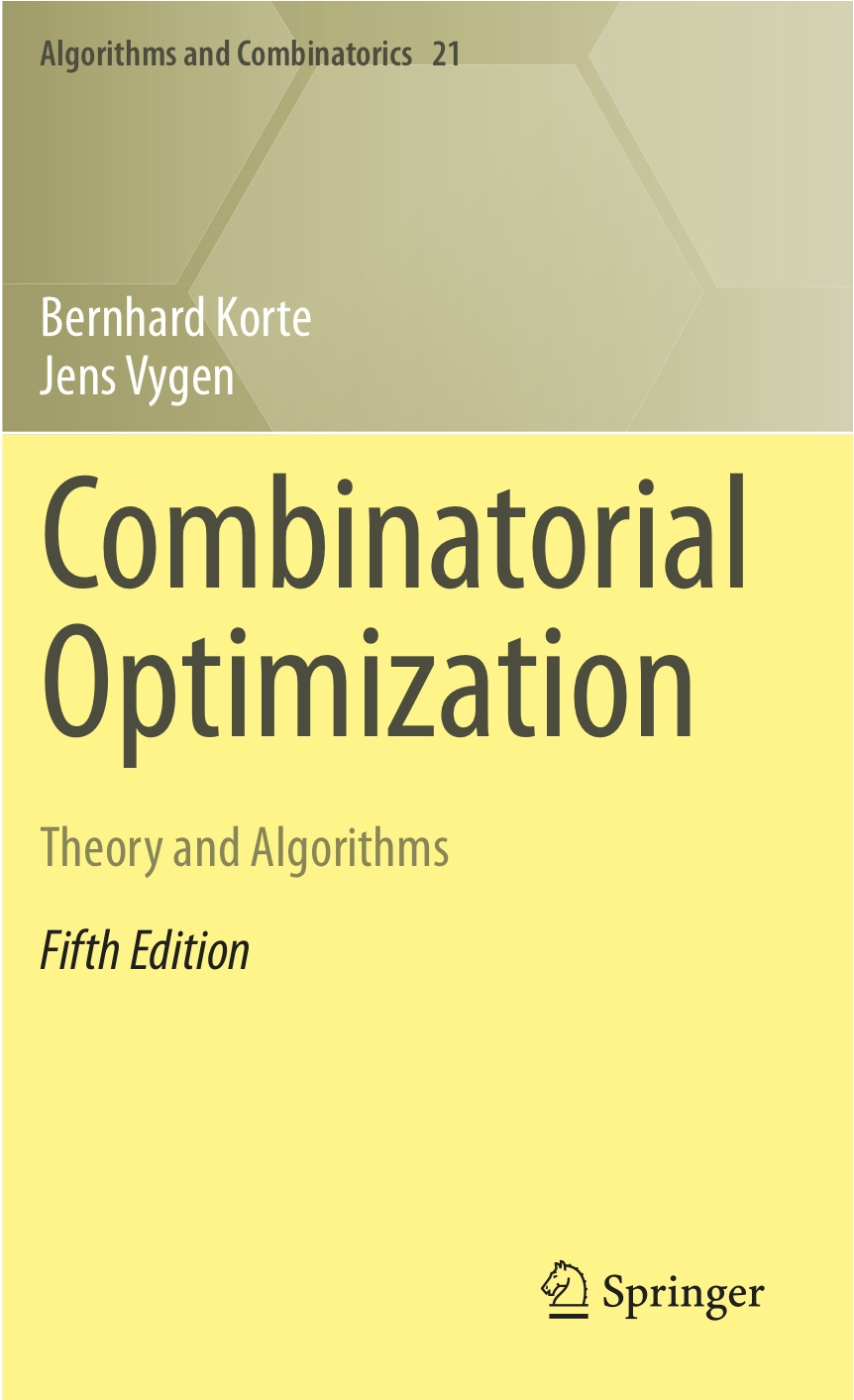# Combinatorial Optimization

## Theory and Algorithms

#### First Edition 2000 Second Edition 2002 Japanese Edition 2005 Third Edition 2006 Fourth Edition 2008 German Edition 2008 Second Japanese Edition 2009 French Edition 2010 Italian Edition 2011 Fifth Edition 2012 Second German Edition 2012 Chinese Edition 2014 (Science Press China) Russian Edition 2015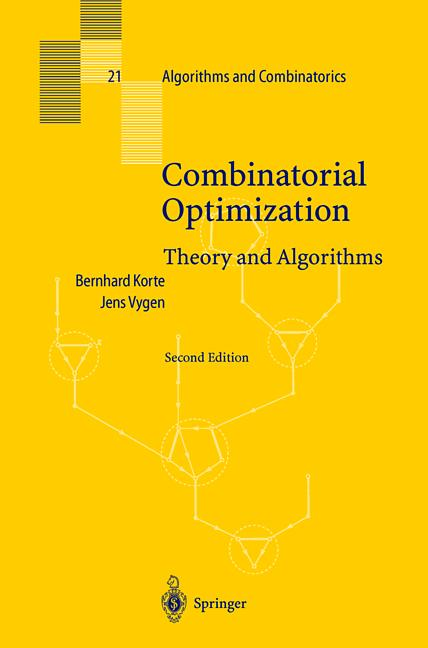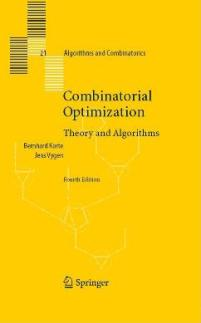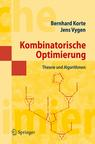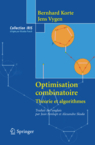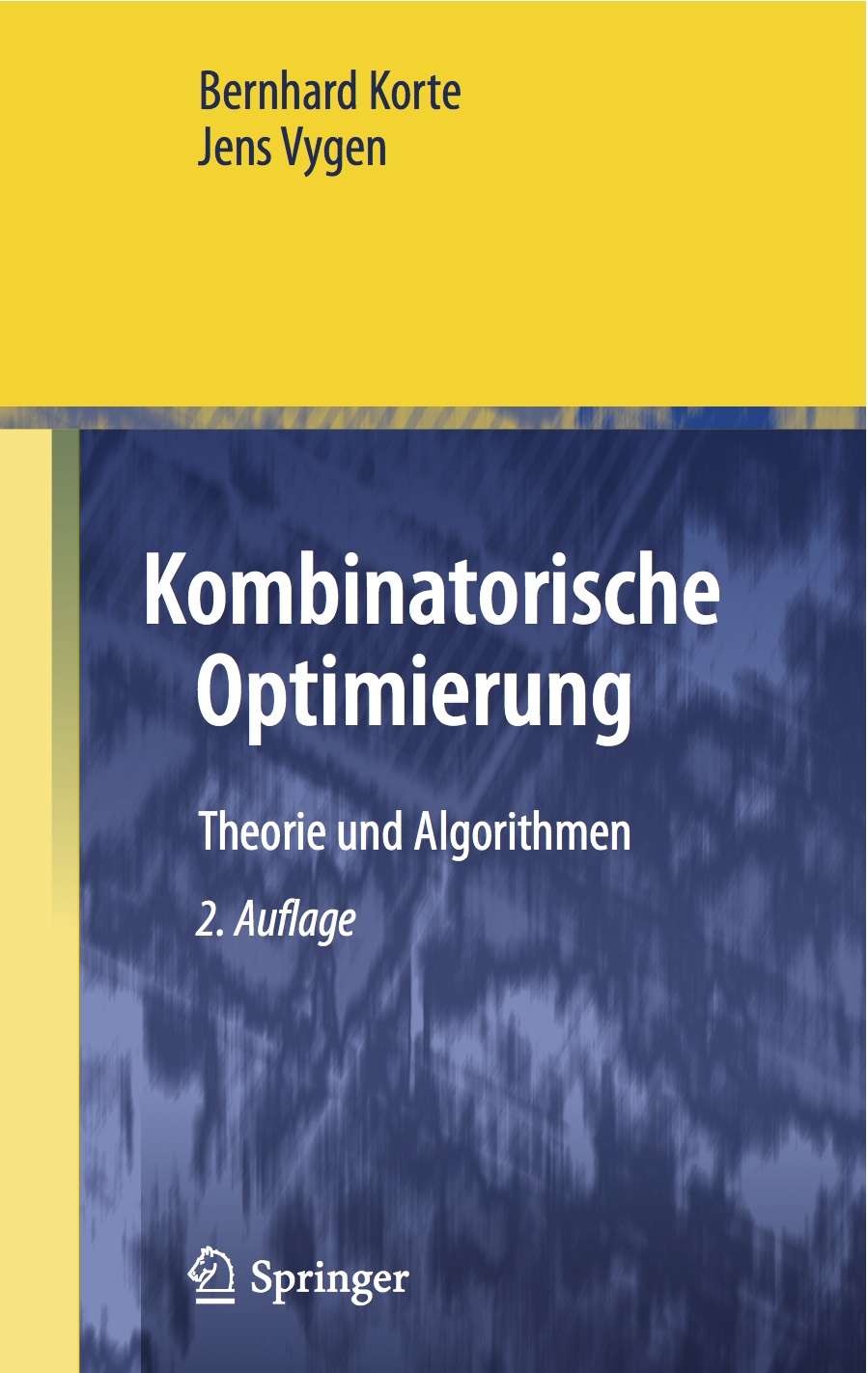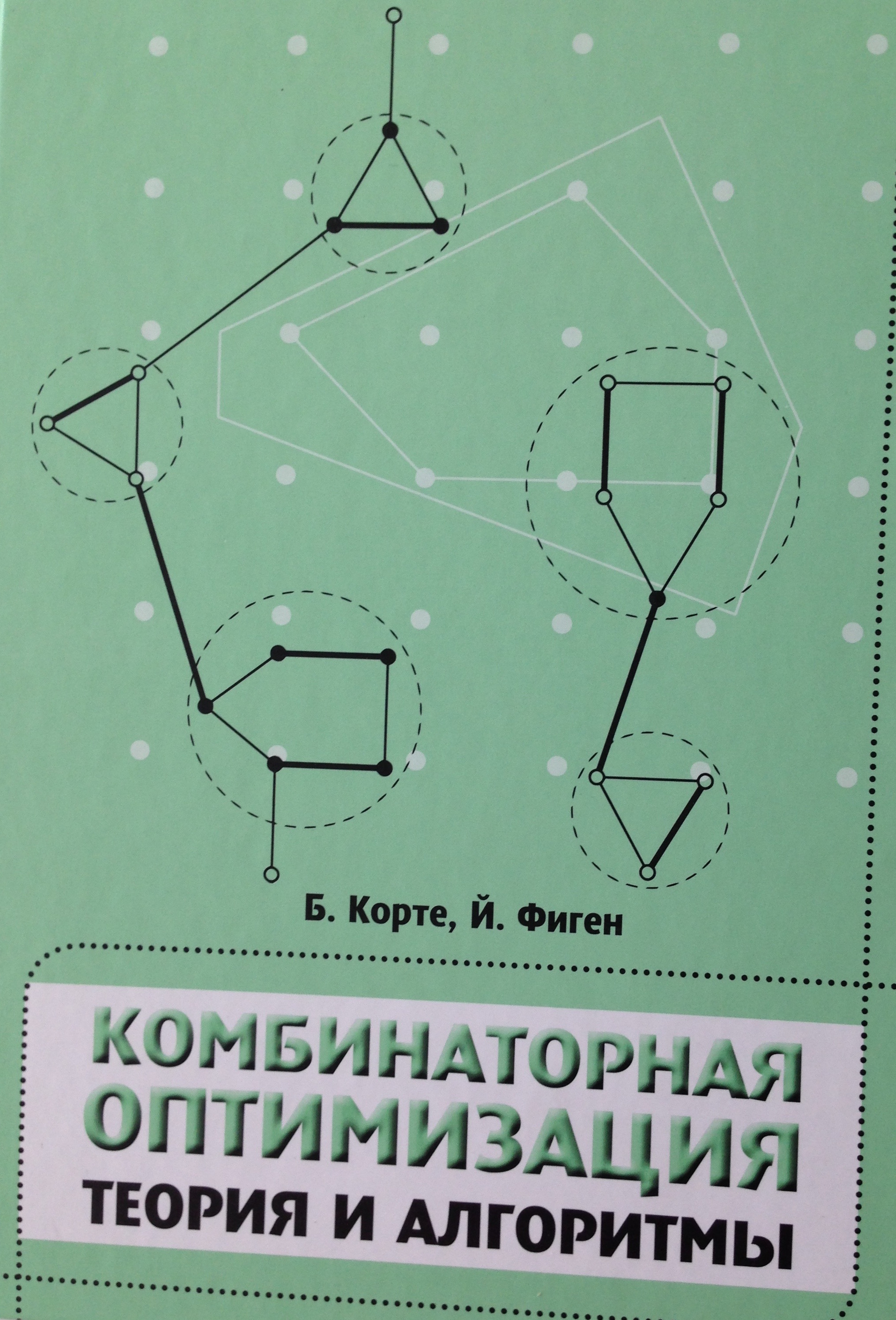# List of Updates and Errors of the Fifth Edition

All entries in the following list refer to the fifth edition. The list is not maintained anymore. (An even older list for the 4th edition is here.) Any additional comments are welcome.

Page Line Comment
18 41 We assume, w.l.o.g., that E(P) is not a subset of E(Q) (otherwise exchange P and Q).
32 4 Replace W_1 by v_1.
32 11 Replace v by v_1.
124 2 Replace $C=\{...\}$ by $C$. The rows of the matrix $A$ in the Hint are $a_1,...,a_t$.
127 36-39 The paper by Dadush, Dey and Vielma  appeared in Mathematical Programming A 145 (2014), 327-348.
137 14-15 During DELETEMIN, one should take only vertices $u$ with $\delta^-(u)=\emptyset$ into consideration.
163 17 Chan's running time was improved by Han and Takaoka  to $O(n^3 \log\log n / \log^2 n)$. Reference: Han, Y., and Takaoka, T. : An O(n^3 log log n / log^2 n) time algorithm for all pairs shortest paths. Journal of Discrete Algorithms 38-41 (2016), 9-19
186 26 Orlin  found an $O(mn)$-time algorithm for the Maximum Flow Problem. Reference: Orlin, J.B. : Max flows in $O(nm)$ time, or better. Proceedings of the 45th Annual ACM Symposium on Theory of Computing (2013), 765-774.
197 4-7 Kawarabayashi and Thorup  showed how to determine the edge-connectivity of a given graph in $O(m\log^{12}n)$ time. Reference: Kawarabayashi, K., and Thorup, M. : Deterministic global minimum cut of a simple graph in near-linear time. Proceedings of the 47th Annual ACM Symposium on Theory of Computing (2015), 665--674.
204 13 Exercise 35 works only for simple graphs.
206 40 The correct page numbers of the paper by Cheung, Lau and Leung  are 197-206.
225 11 Replace "each by less than 2γ" by "adding up to less than 2γ(n-1)".
245 27 The coordinates should be independent.
267 1 Replace k by n.
268 26-30 Exercise 26 does not work; it is not clear how to update $\varphi$ fast enough.
298 41-43 A full version of Gabow's  paper was published on arXiv:1611.07541.
346 28 Condition (b) should be weakened (for the application in the following proof): we need c(xi)>c(yj) for i<j and c(xi)≥c(yj) for i>j.
347 26 Replace x_1,...,x_l by x_l,...,x_1 and y_0,...,y_{l-1} by y_{l-1},...,y_0.
362 30 The proof should begin with the following: Without loss of generality, $f(\emptyset) = g(\emptyset)$ and $f(E) = g(E)$.
369 14 Lee, Sidford and Wong  found a strongly polynomial-time algorithm that minimizes a submodular function in $O(\gamma n^3 \log^2 n + n^4 \log^{O(1)} n)$ time. Reference: Lee, Y.T., Sidford, A., and Wong, S.C.: A faster cutting plane method and its implications for combinatorial and convex optimization. Proceedings of the 56th Annual Symposium on Foundations of Computer Science (2015), to appear
371 23 The algorithm finds a maximal element $F$ of $\mathcal{F}$ with $c(F)$ maximum.
372 13 c should be strictly positive in Exercise 8.
378 -3,-2 Replace Ĺ by Φ. Same on page 379, lines 2, 8, and 34, page 381, line 2, page 384, line 31, page 390, lines 4 and 20, page 391, line 18, and page 407, line -3.
457 29-31 The paper by Singh and Lau appeared in the Journal of the ACM 62 (2015), Article 1.
475 3-9 Dósa and Sgall  improved upper and lower bound of Theorem 18.6 to $\lfloor\frac{17}{10}OPT(I)\rfloor$. Reference: Dósa, G. and Sgall, J.: First fit bin packing: a tight analysis. Proceedings of the 30th International Symposium on Theoretical Aspects of Computer Science (2013), 538--549
486 36-39 The full version of the paper by Dósa  appeared as follows: Dósa, G., Li, R., Han, X., and Tuza, Z.: Tight absolute bound for First Fit Descreasing bin-packing: $\textsl{FFD}(L)\le 11/9\, \textsl{OPT}(L) + 6/9$. Theoretical Computer Science 510 (2013), 13--61
499 23 Replace $z_e$ by $z(e)$.
503 14 Replace $O(|E(H)|)$ by $O(\log |E(H)|)$.
511 30,32 Replace |\delta_H| by |\delta_G| in line 30 and |\delta_H(X)| by |\delta_G(Y)| in line 32.
519 4 The paper by Kawarabayashi, Kobayashi and Reed  appeared in the Journal of Combinatorial Theory B 102 (2012), 424-435.
523 37-38,40 The definition of $q(U\cup\{x\},x)$ is not good, as Lemma 20.4(a) may not hold in general. To fix this, one can either require (w.l.o.g.) $c$ to be a metric or, easier, redefine $q$ as follows: $q(U\cup\{x\},x) := \min \{ c(E(S'))+c(E(S'')) : \emptyset \not= U'\subset U, S' is a Steiner tree for U'\cup\{x\} in G, S'' is a Steiner tree for (U\setminus U')\cup\{x\} in G \}$. Then (a) is trivial, and the proof of (b) is essentially unchanged.
526 30-32 The Steiner tree inapproximability bound was improved to 1.01 by Chlebík and Chlebíková . Reference: Chlebík, M., and Chlebíková, J.: The Steiner tree problem on graphs: Inapproximability results. Theoretical Computer Science 406 (2008), 207-214
529 15-20 The proof of the first statement is not entirely clear, but it actually shows the stronger statement that there exists a spanning tree $M$ in $G[S]$ with $\sum_{\{s,t\}\in E(M)} dist_{(Y,c')}(s,t) \le c(E(Y))-c(L)$.
549 30-31 Replace ≤ by ≥ and one δG(A) by δG(B) in both lines.
553 2 Replace 0 by ∅.
554 19-21 The paper by Byrka et al.  appeared in the Journal of the ACM 60 (2013), Article 6, with the title: Steiner tree approximation via iterative randomized rounding.
556 6 The correct title of Kou's  paper is "On efficient implementation of an approximation algorithm for the Steiner tree problem".
562 6-7 The TSP inapproximability bound was improved to 123/122 by Karpinski, Lampis and Schmied . Reference: Karpinski, M., Lampis, M., Schmied, R. : New inapproximability bounds for TSP. Algorithms and Computation; Proceedings of ISAAC 2013; LNCS 8283 (L. Cai, S.-W. Chen, T.-W. Lam, eds.), Springer, Berlin 2013, pp. 568-578
590 22-25 The paper by Asadpour et al.  appeared in Operations Research 65 (2017), 1043-1061. Svensson, Tarnawski and Végh found a constant-factor approximation algorithm (arXiv:1708.04215).
591 5-7 The paper by Englert, Röglin and Vöcking  appeared in Algorithmica 68 (2014), 190-264.
591 13-14 The paper by Fiorini et al.  appeared in the Journal of the ACM 62 (2015), Article 17, entitled "Exponential lower bounds for polytopes in combinatorial optimization".
617 7 "element of" should be "subset of".
627 42-46 The paper by Levi, Shmoys and Swamy  appeared in Mathematical Programming A 131 (2012), 365-379.
627 47-50 The paper by Li  appeared in Information and Computation 222 (2013), 45-58.

Last change: September 5, 2017. Thanks to Maxim Babenko, Steffen Böhmer, Ulrich Brenner, György Dósa, Michael Etscheid, Jean Fonlupt, Stephan Held, Stefan Hougardy, Solomon Lo, Jens Maßberg, Dieter Rautenbach, Jan Schneider, Sophie Spirkl, and Uri Zwick.